# Atomic Structure

Structure of an Atom

• Atoms are the smallest units of an element.
• Atoms are made of sub-atomic particles:
• protons (relative charge +1, relative mass 1)
• neutrons (relative charge 0, relative mass 1)
• electrons (relative charge -1, relative mass 1/1840)
• The number of protons an atom has is called its ‘atomic number’.
• The centre of an atom is called a nucleus; it contains just protons and neutrons, making the nucleus positively charged.
• Electrons are attracted to the positive charge of a nucleus and exist in orbitals around the nucleus.
• Atoms can lose or gain electrons to become charged ions.

Electron Orbitals

• Electrons are negatively charged, so they are attracted to the positive charge of a nucleus (electrostatic attraction).
• The closer an electron is to a nucleus, the lower its energy.
• All electrons in an atom want to get as close to the nucleus as possible, but they repel each other.
• This forces electrons to exist in set regions called orbitals.
• One orbital can hold two electrons (one pair).
• Different orbitals have different shapes and energies. Orbital types are labelled with letters – s, p, d and f.
• Shells describe how far away an electron is from a nucleus, and therefore its energy.
• Shell 1 is the closest shell to the nucleus, shell 2 the next closest, etc.
• Due to different shapes, orbitals within a shell can have different energies and form sub-shells.
• Shells and sub-shells are filled successively – lowest available energy sub-shells and orbitals are filled first.

Electron Configurations

• Electrons occupy orbitals of lowest energy around a nucleus.
• Higher energy orbitals are only occupied if all lower energy orbitals are already full.
• Electron configurations show how electrons are arranged around a nucleus within an atom or ion.
• The first number shows the shell the electrons are in.
• The letter shows the shape of the orbital the electrons are in.
• The second number shows the number of electrons in the orbital(s).
• Due to electron repulsion and orbital shapes, 4s orbitals are occupied before the 3d orbitals.

# Moles (Amounts)

The Mole

• Atoms and particles are very small, so referring to actual number of atoms in a sample isn’t very practical.
• The mole is a unit used to refer to an amount of atoms or particles.
• Just like one dozen = 12 of something, one mole = 6.022 x 1023 of something.
• The number one mole refers to is known as Avogadro’s constant.

Relative Atomic Mass

• Relative atomic masses (Ar) are used to compare the masses of all elements on the periodic table.
• Relative atomic mass (Ar) is the weighted average mass of one atom of each element – compared to 1/12th the mass of one atom of carbon-12.
• one carbon-12 atom has six protons and six neutrons
• relative mass of one proton is one
• relative mass of one neutron is one
• relative mass of one atom of carbon-12 is twelve
• The mass of an atom is determined by the number of protons and neutrons it has.
• Protons and neutrons have (virtually) the same mass.
• The actual mass of protons and neutrons are too small to use when describing the mass of an atom, instead both particles are assigned a relative mass of one.
• Relative atomic mass is a weighted average that accounts for the abundance of each naturally occurring isotope of that element.
• Molar mass is the mass (g) of one mole’s worth of something (g mol-1).

Moles from Mass

• The number of moles in a sample can be calculated using the formula: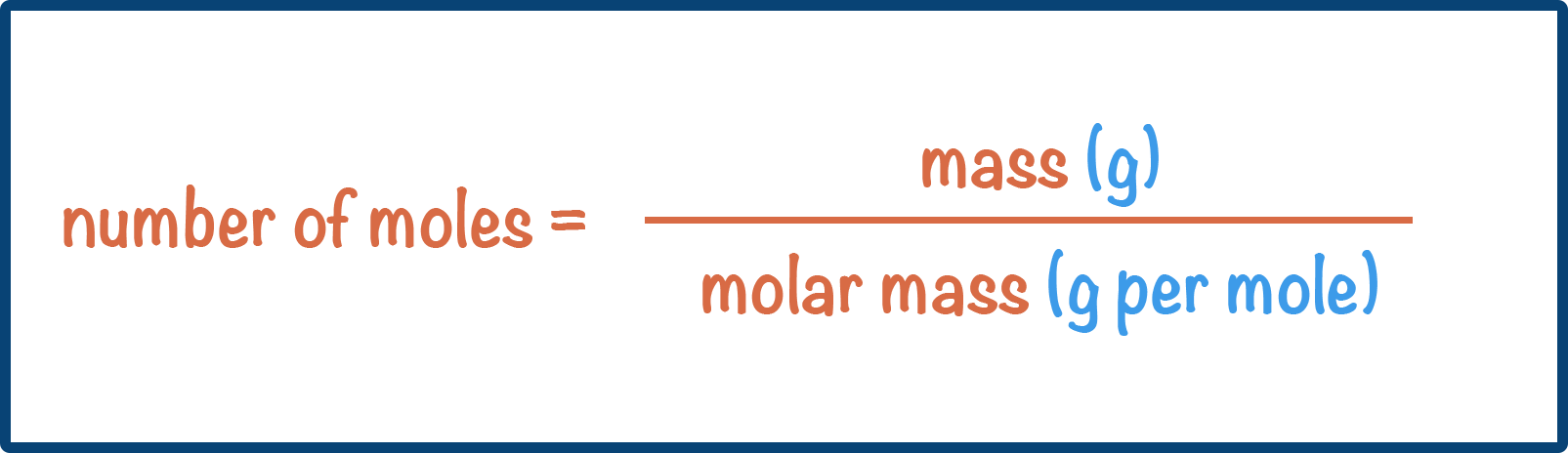• The value of relative atomic mass of an element = value of molar mass (in grams per mol).
• The value of relative formula and molecular mass = value of molar mass (in grams per mol).
• This means moles can be calculated using: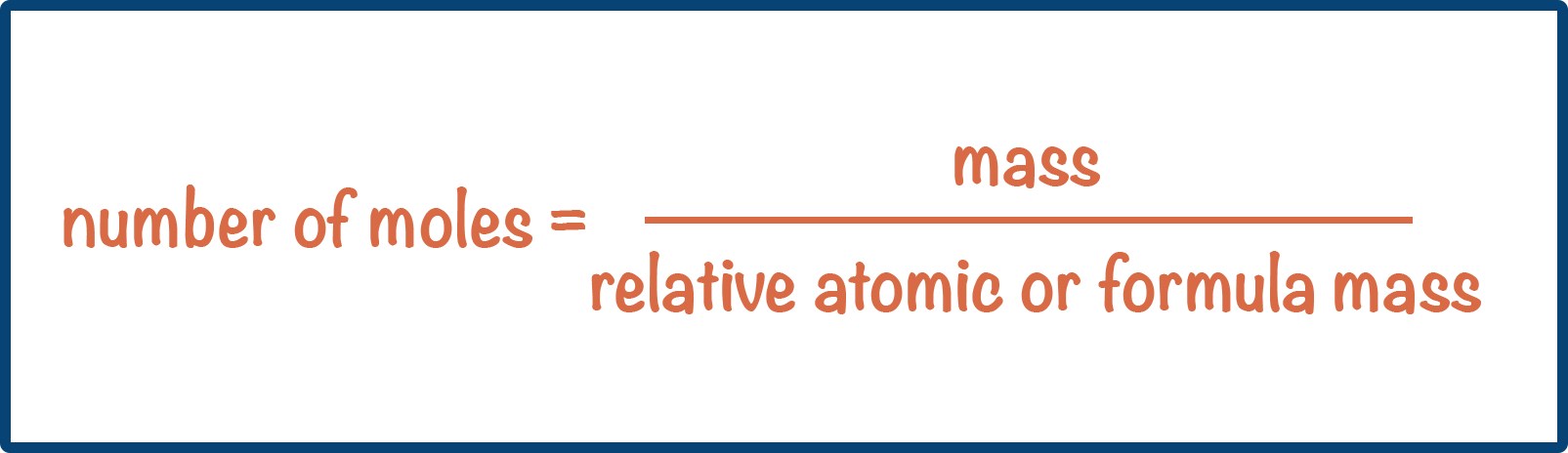Moles - Solutions

• The number of moles of a solute in a solution can be calculated using the formula: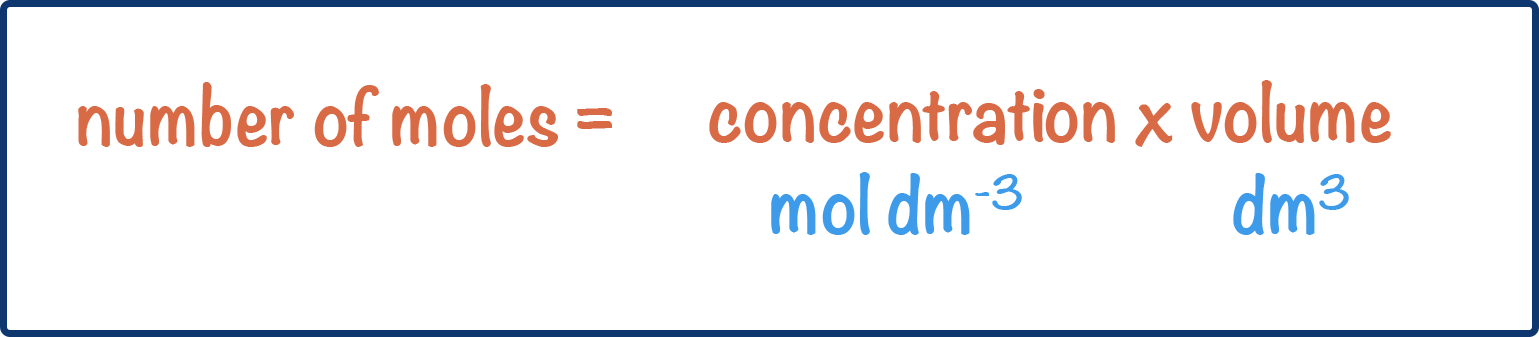• Volume (of solution) is usually given in cm3 and must be converted into dm3.
• 1 dm3 is the same as 1000 cm3
• To convert dm3 to cm3 x 1000
• To convert cm3 to dm3 / 1000
• Concentration refers to how many moles of solute are in 1dm3 of solution (units are mol dm-3).

Moles - Gases

• The ideal gas equation is used to calculate the moles of gas present in a system if the volume, pressure and temperature are known.
• pV = nRT
• n = moles
• R = gas constant = 8.31 Jmol-1K-1
• V = volume (m3)
• p = pressure (Pa)
• T = temperature (K)
• The equation assumes that all gases behave in the same way and are not interacting with each other, they are described as ‘ideal gases’.
• At room temperature and pressure (293K and 101kPa), the ideal gas equation shows that 1 mole of any gas occupies a total volume of 24000cm3 (24dm3).
• At standard temperature and pressure (273K and 101kPa), the ideal gas equation shows that 1 mole of any gas occupies a total volume of 22400cm3 (22.4dm3).

# Bonding

Bonding

• Bonds between atoms are called atomic bonds.
• Forces between molecules are called intermolecular forces.
• Intermolecular forces are broken and formed when molecular substances change state (solid, liquid and gas).

Covalent Bonding

• A covalent bond is the sharing of one pair of electrons between two atoms.
• Two atomic orbitals from two atoms overlap and create a new area of electron density between the two atoms, called a molecular orbital.
• The positively charged nuclei of both atoms get attracted to the shared pair of negatively charged electrons and this pulls them closer together.• The distance between the centre of each nuclei to each other is called a ‘bond length’.
•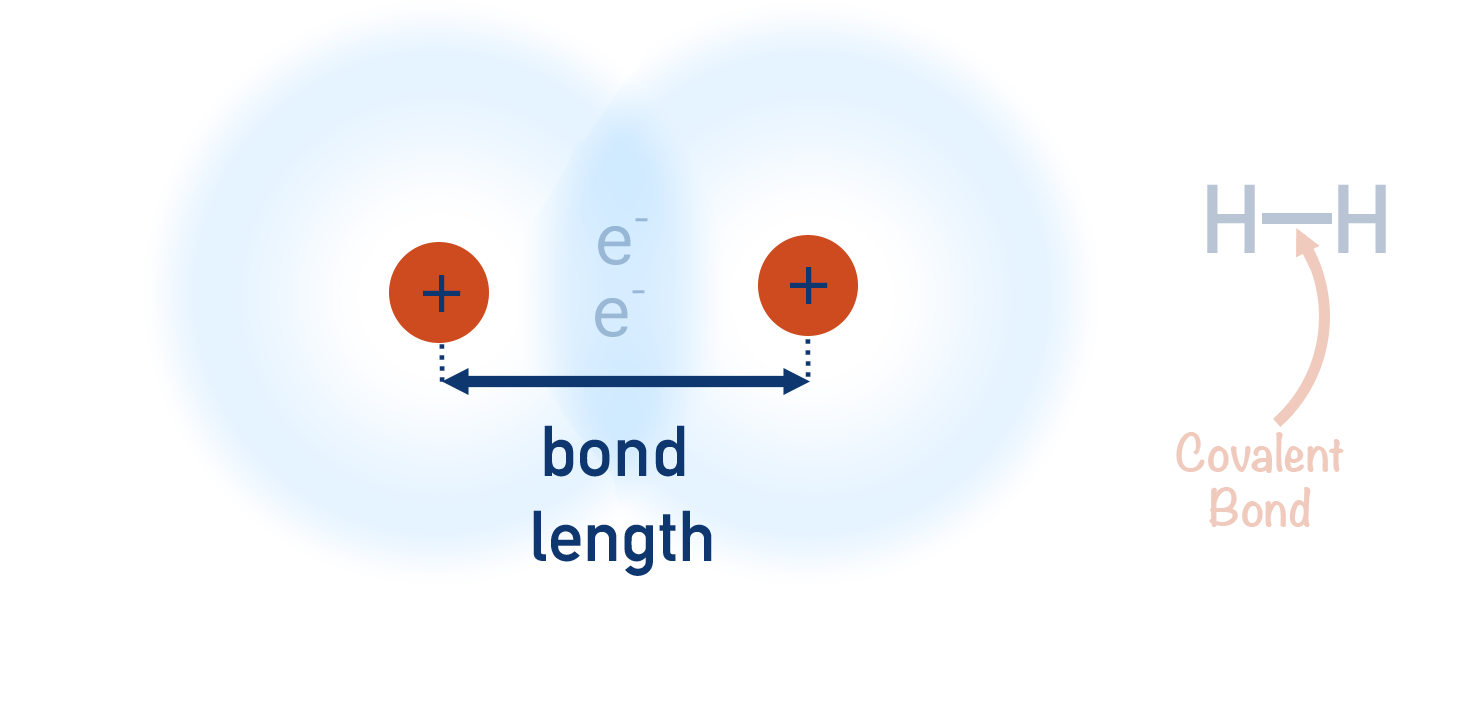• Non-metals form covalent bonds with other non-metals.
• As covalent bonds occur between atoms, they are atomic bonds.

Electronegativity

• Electronegativity is the ability or 'tendency' of an atom to attract a shared pair of electrons in a covalent bond towards itself.
• If two atoms with different electronegativities are covalently bonded together, the bond will be polar.
•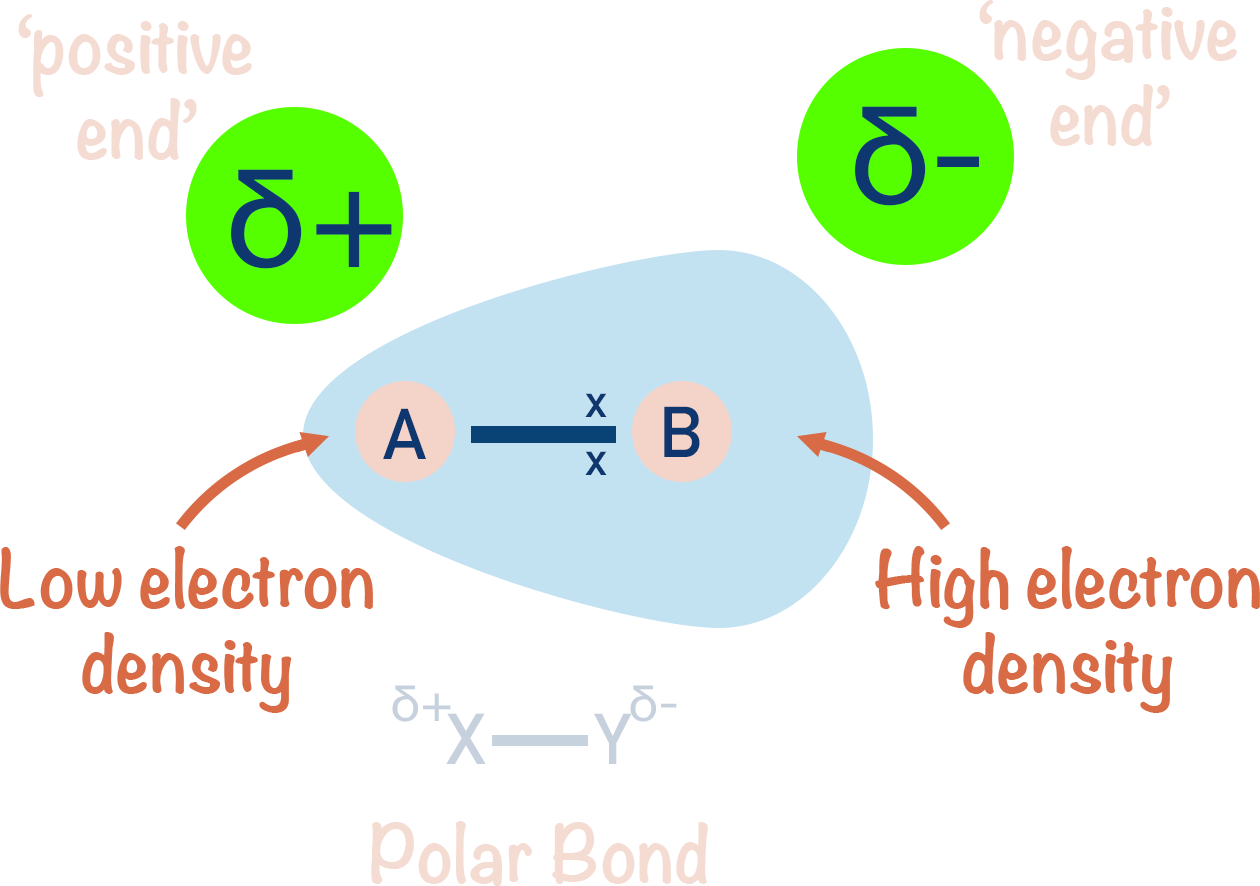• The atom with the higher electronegativity will attract the bonded electrons towards itself and will end up with a partial negative charge (δ-).
• The atom with the lower electronegativity will have the bonded electrons pulled away from it and will end up with a partial positive charge (δ+).
• The greater the difference in electronegativity between two bonded atoms, the more polar the bond.
• Electronegativity increases across a period in the periodic table and decreases down a group.
• Electronegativities are relative and the Pauling Scale is often used to compare the electronegativities of different elements - Francium has the lowest electronegativity (0.7) and Fluourine the highest (4.0).

Ionic Bonding

• Ionic bonding describes the electrostatic attraction that holds oppositely charged ions together.
• The strength of an ionic bond is based on the charge and size of the ions involved:
• Greater the charge = stronger the bond.
• Smaller the ionic radius = stronger the bond
• To get a stable electron configuration, atoms lose or gain electrons.
• Metals lose electrons to get a full outer shell and become positively charged ions.
• Non-metals (usually) gain electrons to get a full outer shell and become negatively charged ions.
• Oppositely charged ions arrange themselves in a solid to maximise attraction of opposite charges and minimise repulsion to like charges, forming a repeating lattice structure.
•Metallic Bonding

• In metallic bonding, positive metal ions are attracted to negatively charged delocalised electrons, forming a dense and rigid structure.
•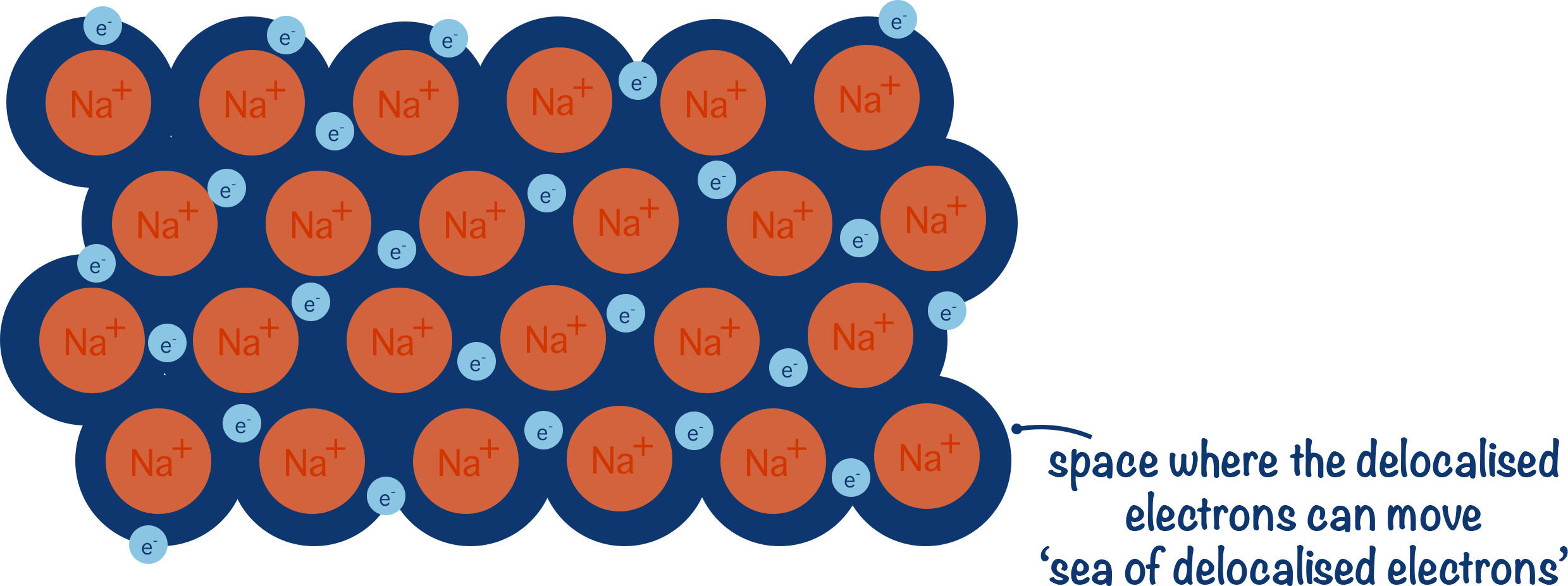• Positively charged metal ions form because outermost electrons are held weakly by the metal atoms nucleus and are able to drift out of their orbitals, becoming delocalised and leaving a positively charged metal ion behind.
• Delocalised electrons from metal atoms have 'nowhere to go' and stay attracted to the positive charge of metal ions.
• Electrons are not held by the metal atom but are free to move – they are delocalised.
• Free movement of delocalised electrons enable metals to conduct electricity.

Intermolecular Forces

• Intermolecular forces hold molecules together in molecular substances.
• Temporary induced dipole-dipole forces (London dispersion forces) exist between all molecules.
• Unequal electron distribution around a molecule creates an instantaneous dipole that can induce a dipole on a neighbouring molecule.
• Two opposite dipoles from different molecules are attracted to each other and a weak force occurs.
• They are the weakest type of intermolecular force.
• The bigger the molecules, the greater the intermolecular forces that can arise between them, giving larger molecules higher melting and boiling points.
• Partial charges are shown using δ+ (partially positive) and δ- (partially negative).

Permanent Dipole-Dipole

• Permanent dipole-dipole interactions exist between polar molecules.
• The partially negatively charged area of one molecule is attracted to a partially positively charged area of another molecule.
• The attractions are permanent and are stronger than temporary, induced dipole-dipole forces.
• The greater the polarity of a bond or molecule, the stronger the permanent dipole-dipole interactions.

Hydrogen Bonding

• Hydrogen bonds are a specific type of intermolecular force.
• Molecules that can form hydrogen bonds have higher melting and boiling points than similiar sized molecules that are unalbe to.
• Hydrogen bonds exist between molecules that have a N-H, O-H or F-H bond.
• Nitrogen, oxygen and fluorine are the most electronegative elements, so the covalent bonds between them and hydrogen are highly polar.
• Highly polar bonds result in strong attractive forces between molecules.
• The partially negative nitrogen, oxygen or fluorine forms hydrogen bonds with the partially positive hydrogen atoms from other molecules.
• Substances that can form hydrogen bonds have higher melting points than similar-sized substances that cannot.

# Energy and Enthalpy

Enthalpy Changes

• During chemical reactions energy is exchanged between reacting substances and their surroundings.
• Exothermic reactions – products are lower in energy than the reactants and the temperature of the surroundings increases.
• Endothermic reactions – products are higher in energy than the reactants and the temperature of the surroundings decreases.
• Enthalpy is a measure of heat energy with the units kJmol-1.
• The actual amount of energy in a compound or atom is not easily measured in chemistry, so it is the change in heat energy during a reaction (enthalpy change, ΔH) that is measured.
• Exothermic reactions have negative enthalpy changes (-ΔH).
• Endothermic reactions have positive enthalpy changes (+ΔH).
• Standard enthalpy change refers to the thermal energy change that occurs during a reaction, where 1 mole of product is formed in standard conditions (101 kPa and 298K).

Bond Enthalpies

• The overall enthalpy change that occurs in a reaction can be found by subtracting the sum of all the bond enthalpies of bonds formed from the sum of all bond enthalpies of bonds broken.
• A bond enthalpy is the amount of energy released or absorbed when a particular bond is made or broken.
• Energy is released by two atoms when a bond is formed between them.
• That same amount of energy is absorbed by the two atoms when the bond is broken.
• Mean bond enthalpies are used to account for the different environments a bond might be in.

Calorimetry

• Calorimetry is a technique used to find the heat energy change that occurs during a reaction.
• Heat energy change is found by measuring how the temperature of the substance surrounding the reaction changes.
• Specific heat capacity (c) refers to the energy required to raise the temperature of 1g (or 1kg) of a substance by 1oC.
• The temperature change of a substance can be used with its specific heat capacity to find the energy it has absorbed or released.
• Change in energy = mass x specific heat capacity x change in temperature
• Q = mcΔT
• Energy is often lost from the substance, whose temperature is being measured, to the surroundings.
• To increase the accuracy of calorimetry, heat loss must be minimised (extra insulation can help).

Hess' Law

• Hess’ Law states that the energy change that occurs when a substance is changed into a product is the same, regardless of the route taken.
• There is often more than one way to go from a reactant to a product, meaning there is more than one ‘route’ to convert a substance to a particular product.
• Hess cycles are ways of drawing out the energy changes that occur when a substance is turned into a different substance via more than one route.

# Kinetics

Collision Theory

• Kinetics is the study of how fast reactions occur.
• How quickly a reaction happens is called its reaction rate.
• Reactions occur when reactant particles collide together with enough energy (activation energy, Ea).
• Collisions between particles that lead to a reaction are called ‘successful collisions’.
• Increasing the frequency of successful collisions increases the rate of reaction.
• Increasing the temperature, concentration and pressure of reacting particles increases the rate of a reaction.

Boltzmann Distribution Curves

• Maxwell-Boltzmann distribution curves show how available energy is distributed among molecules in a gaseous system.
• At a given temperature, only a certain amount of energy is available for all molecules of gas within a system.
• Individual molecules have different energies and are constantly colliding, transferring energy with other molecules – either gaining or losing energy.
• The effects of changing concentration, temperature and the use of a catalyst on the energies of particles can be shown.

Equilibrium

• In a reversible reaction, reactants react to produce products (forward reaction) and those products also react to produce the original reactants (reverse reaction).
• At equilibrium, the rates of both the forward and reverse reactions are the same – the concentrations of all reactants and products do not change.
• The position of equilibrium describes how much one reaction has been favoured compared to the other before equilibrium is reached.
• Changing the conditions of an equilibrium system can cause the position of equilibrium to move.
• Le Chatelier’s Principle states that the position of equilibrium will move to oppose a change in conditions.
• Increasing the temperature of a system forces the position of equilibrium to move to favour the endothermic reaction.
• Increasing the concentration of a reactant forces the position of equilibrium to move to favour the reaction that uses up that reactant.
• Increasing the pressure of a system forces the position of equilibrium to move to favour the reaction that produces the fewest moles of gas.